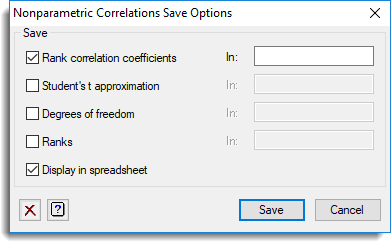1. Home
2. Nonparametric Correlation Save Options

# Nonparametric Correlation Save Options

Use this to save results from Spearman’s rank correlation or Kendall’s rank correlation coefficient.

1. After selecting the appropriate boxes, type names for the data structures into the corresponding In: fields.## Save

The tables below indicate the type of structures formed for each item.

### Spearman’s rank correlation

 Rank Correlation coefficients Scalar or symmetric matrix Saves the correlation coefficient for each pair of samples. Student’s t approximation Scalar or symmetric matrix Saves the Student’s t approximation to the correlation coefficient for each pair of samples. Degrees of freedom Scalar or symmetric matrix Saves the degrees of freedom for each t statistic. Ranks Pointer Saves the ranks of each sample.

### Kendall’s rank correlation coefficient

 Rank correlation coefficients Scalar or symmetric matrix Saves the correlation coefficient for each pair of samples. Probabilities Scalar or symmetric matrix Saves the probability to the correlation coefficient for each pair of samples. Normal approximation Scalar or symmetric matrix Saves the Normal approximation to the correlation coefficient for each pair of samples.